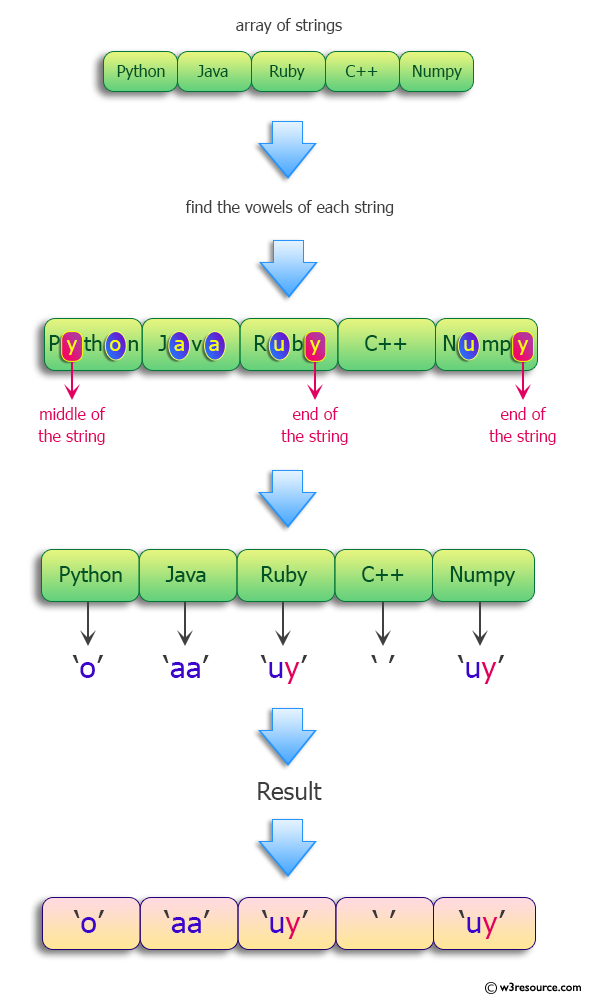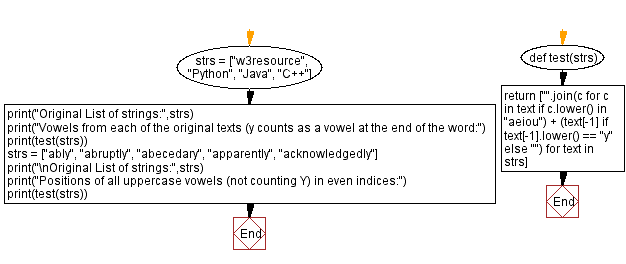﻿ Python: Find the vowels from each of the original texts (y counts as a vowel at the end of the word) - w3resource# Python: Find the vowels from each of the original texts (y counts as a vowel at the end of the word)

## Python Programming Puzzles: Exercise-86 with Solution

Write a Python program to find the vowels from each of the original texts (y counts as a vowel at the end of the word) from a given list of strings.

```Input:
['w3resource', 'Python', 'Java', 'C++']
Output:
['eoue', 'o', 'aa', '']

Input:
['ably', 'abruptly', 'abecedary', 'apparently', 'acknowledgedly']
Output:
['ay', 'auy', 'aeeay', 'aaey', 'aoeey']
```

Pictorial Presentation:Sample Solution:

Python Code:

``````#License: https://bit.ly/3oLErEI

def test(strs):
return ["".join(c for c in text if c.lower() in "aeiou") + (text[-1] if text[-1].lower() == "y" else "")
for text in strs]

strs = ["w3resource", "Python", "Java", "C++"]
print("Original List of strings:",strs)
print("Vowels from each of the original texts (y counts as a vowel at the end of the word:")
print(test(strs))
strs = ["ably", "abruptly", "abecedary", "apparently", "acknowledgedly"]
print("\nOriginal List of strings:",strs)
print("Positions of all uppercase vowels (not counting Y) in even indices:")
print(test(strs))
``````

Sample Output:

```Original List of strings: ['w3resource', 'Python', 'Java', 'C++']
Vowels from each of the original texts (y counts as a vowel at the end of the word:
['eoue', 'o', 'aa', '']

Original List of strings: ['ably', 'abruptly', 'abecedary', 'apparently', 'acknowledgedly']
Positions of all uppercase vowels (not counting Y) in even indices:
['ay', 'auy', 'aeeay', 'aaey', 'aoeey']
```

Flowchart:## Visualize Python code execution:

The following tool visualize what the computer is doing step-by-step as it executes the said program:

Python Code Editor :

Have another way to solve this solution? Contribute your code (and comments) through Disqus.

What is the difficulty level of this exercise?

Test your Programming skills with w3resource's quiz.

﻿

## Python: Tips of the Day

Clamps num within the inclusive range specified by the boundary values x and y:

Example:

```def tips_clamp_num(num,x,y):
return max(min(num, max(x, y)), min(x, y))
print(tips_clamp_num(2, 4, 6))
print(tips_clamp_num(1, -1, -6))
```

Output:

```4
-1
```